Jump to content

# Linear Transformations: Polynomial to its second derivatives

## Recommended Posts

Consider the Vector Space of Polynomial with degree <= 5.
And the transformation to its second derivatives.
Shows the transformation and its matrix em relate to the base of this space.

Could anybody help me?
Thanks!

##### Share on other sites

You could choose a basis for the space of polynomials over $$\mathbb{R}$$ in a variable $$x$$ of degree at most 5.

A 'random' such basis might be $$\{ 1 + x^3 -x^5, x + x^2- x^3, x^2 +x^4, x^3 + x^5, x^4 - x^5, 2x^5\}$$.

How does your transformation act on the elements of this basis?

If any polynomial can be expressed as a linear combination of the polynomials in the basis, how would the transformation act on it?

Can you find an even better basis to use to show the same?

How will the transformation look in matrix notation?

Edited by taeto
##### Share on other sites

Depends on how you order your basis. Let's say {1,x,x2,...} (I'd pick a 'natural' basis, meaning one in which your matrix looks simpler, of course any non-singular linear combo of them would do).

The transform of xn is n(n-1)xn-2, so what it does is to multiply by n(n-1) and shift it twice to the right (here's where the ordering of you basis matters in what the matrix looks like, so if you order the other way around, the T --transformation-- matrix would look like the transpose).

So your matrix would be something like,

That is,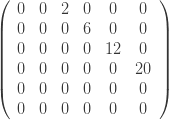Please, check. I may have made some ordering mistake, missed a row, etc.

Edited by joigus
bad rendering of image (formula)
##### Share on other sites

That looks right.

Then a polynomial in your space is represented as a vector.

And the transformation maps this vector to another vector using matrix multiplication, or does it?

##### Share on other sites
52 minutes ago, taeto said:

That looks right.

Then a polynomial in your space is represented as a vector.

And the transformation maps this vector to another vector using matrix multiplication, or does it?

Exactly. 1 would be (1 0 0 0 0 0), x: (0 1 0 0 0 0), x2: (0 0 1 0 0 0), etc. (read as column vectors).

And d/dx of (0 0 1 0 0 0) is (2 0 0 0 0 0) just as d/dx of x2 is 2 times 1.

##### Share on other sites

Would it be possible to say that differentiation $$\frac{d}{dx}$$ can be represented as a matrix $$M$$, and that second derivative $$\frac{d^2}{dx^2}$$ can be represented by the squared matrix $$M^2$$?

##### Share on other sites
18 minutes ago, taeto said:

Would it be possible to say that differentiation ddx can be represented as a matrix M , and that second derivative d2dx2 can be represented by the squared matrix M2

Exactly right. Check it yourself. It's a fun exercise. On that space, the diff operator "is" the matrix.

##### Share on other sites

There is probably a theorem somewhere to say that it does work out that way, just because that is how linear functions work when you compose them. Their matrices get multiplied together.

So if this works for polynomials of degree at most 5, it should work the same for polynomials of any bounded degree d. All you need is to use matrices with d+1 rows and columns to represent differentiation, and then their k'th powers represent k times differentiation for k < d.

But polynomials can have all kinds of degrees. The next question is how to deal with the linear transformation of differentiating a polynomial when we do not know how large its degree might be. If you have a 100x100 matrix to differentiate any polynomial of degree < 100, then it doesn't work to differentiate a polynomial of degree 100 or more.

##### Share on other sites
1 hour ago, taeto said:

There is probably a theorem somewhere to say that it does work out that way, just because that is how linear functions work when you compose them. Their matrices get multiplied together.

So if this works for polynomials of degree at most 5, it should work the same for polynomials of any bounded degree d. All you need is to use matrices with d+1 rows and columns to represent differentiation, and then their k'th powers represent k times differentiation for k < d.

But polynomials can have all kinds of degrees. The next question is how to deal with the linear transformation of differentiating a polynomial when we do not know how large its degree might be. If you have a 100x100 matrix to differentiate any polynomial of degree < 100, then it doesn't work to differentiate a polynomial of degree 100 or more.

You're right, there is a theorem. It's really to do with the fact that you've got a linear isomorphism, that is, a mapping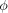such that,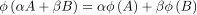that is, that preserves the linear operations in your initial space. Your initial space must be a linear space too under (internal) sum and (external) multiplication by a constant.

Now, objects A, B, etc. can be most anything. They can be polynomials, sin cos functions, anything.

The key facts are both that the d/dx operator is linear and the polynomials under sum and product by scalars are a linear space.

Thewould be assigning a vector to a polynomial.

And your intuition is correct. There is no limit to the possible dimension of a linear space. Quantum mechanics, for example, deals with infinite dimension spaces, so the transformation matrices used there are infinite-dimensional matrices. In that case it's not very useful to write the matrices as "tables" on a paper.

I hope that helps.

Edited by joigus
Line added
##### Share on other sites

Do you see whether there is a matrix $$\bar{M}$$ that can map a polynomial to its integral? Since integration followed by differentiation produces the same polynomial that you started from, it should then be that $$M$$ and  $$\bar{M}$$ are inverse matrices, if $$M$$ is the 'differentiation matrix'.

##### Share on other sites

Well, yes, but you must be careful with a couple of things.

First: If you integrate x5 you get off limits. x6 no longer is in your space. You must expand your space so as to include all possible powers.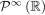. Then you're good to go.

Second: You must define your integrals with a fixed prescription of one limit point. For example,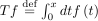so that they are actually 1-valued mappings. Then it's correct.

You don't have this problem with derivatives, as you can derive number zero till you're blue in your mouth and never get off limits.

If you were using functions other than polynomials, you would have to be careful with convergence of your integrals. But polynomials are well-behaved functions in that respect.

Hope it helps.Edited by joigus
Line added
##### Share on other sites
7 hours ago, joigus said:

Exactly. 1 would be (1 0 0 0 0 0), x: (0 1 0 0 0 0), x2: (0 0 1 0 0 0), etc. (read as column vectors).

And d/dx of (0 0 1 0 0 0) is (2 0 0 0 0 0) just as d/dx of x2 is 2 times 1.

Sorry, I meant And d2/dx2 of (0 0 1 0 0 0) is (2 0 0 0 0 0) just as d2/dx2 of x2 is 2 times 1.

## Create an account or sign in to comment

You need to be a member in order to leave a comment

## Create an account

Sign up for a new account in our community. It's easy!

Register a new account

## Sign in

Already have an account? Sign in here.

Sign In Now
×

• #### Activity

• Leaderboard
×
• Create New...

## Important Information

We have placed cookies on your device to help make this website better. You can adjust your cookie settings, otherwise we'll assume you're okay to continue.